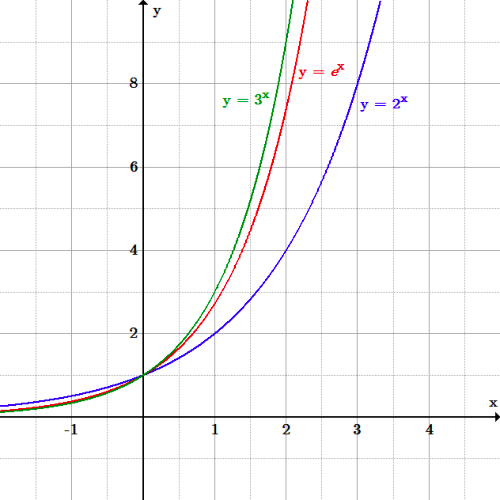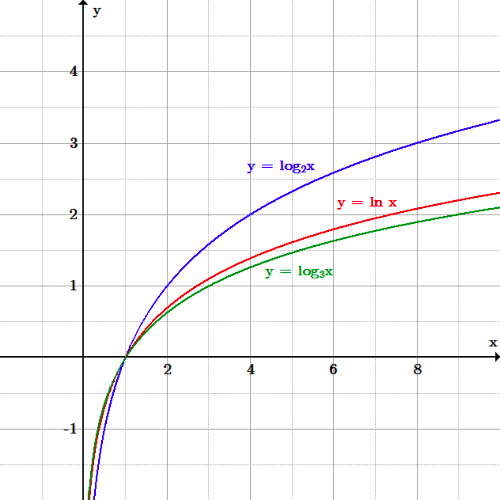The Number e

The number $e$ is an important mathematical constant, approximately equal to $2.71828$. When used as the base for a logarithm, we call that logarithm the natural logarithm and write it as $\ln x$.

Learning Objectives

Recognize the properties and uses of the number $e$

Key Takeaways

Key Points

• The natural logarithm, written $f(x) = \ln(x)$, is the power to which $e$ must be raised to obtain $x$.
• The constant can be defined in many ways, most of which involve calculus. For example, it is the limit of the sequence whose general term is $(1+{1 \over n})^n$. Also, it is the unique number so that the area under the curve $y={1 \over x}$ from $x=1$ to $x=e$ is $1$ square unit.

Key Terms

• e: The base of the natural logarithm, 2.718281828459045…
• logarithm: The logarithm of a number is the exponent by which another fixed value, the base, has to be raised to produce that number.

The Number $e$

The number $e$, sometimes called the natural number, or Euler’s number, is an important mathematical constant approximately equal to 2.71828. When used as the base for a logarithm, the corresponding logarithm is called the natural logarithm, and is written as $\ln (x)$. Note that $\ln (e) =1$ and that $\ln (1)=0$.

There are a number of different definitions of the number $e$. Most of them involve calculus. One is that $e$ is the limit of the sequence whose general term is $(1+{1 \over n})^n$. Another is that $e$ is the unique number so that the area under the curve $y=1/x$ from $x=1$ to $x=e$ is $1$ square unit.

Another definition of $e$ involves the infinite series $1+\frac{1}{1!}+\frac{1}{2!}+\frac{1}{3!}+\frac{1}{4! }+….$. It can be shown that the sum of this series is $e$.

Importance of $e$

The number $e$ is very important in mathematics, alongside $0, 1, i, \, \text{and} \, \pi.$ All five of these numbers play important and recurring roles across mathematics, and are the five constants appearing in the formulation of Euler’s identity, which (amazingly) states that $e^{i\pi}+1=0.$ Like the constant $\pi$, $e$ is irrational (it cannot be written as a  ratio of integers), and it is transcendental (it is not a root of any non-zero polynomial with rational coefficients).

Compound Interest

One of the many places the number $e$ plays a role in mathematics is in the formula for compound interest. Jacob Bernoulli discovered this constant by asking questions related to the amount of money in an account after a certain number of years, if the interest is compounded $n$ times per year. He was able to come up with the formula that if the interest rate is $r$ percent and is calculated $n$ times per year, and the account originally contained $P$ dollars, then the amount in the account after $t$ years is given by $A=P(1+{r \over n})^{nt}.$ By then asking about what happens as $n$ gets arbitrarily large, he was able to come up with the formula for continuously compounded interest, which is $A=Pe^{rt}.$

Graphs of Exponential Functions, Base e

The function $f(x) = e^x$ is a basic exponential function with some very interesting properties.

Learning Objectives

Identify important properties about the graph of $f(x) = e^x$

Key Takeaways

Key Points

• The function $f(x)=e^{x}$ is a function which is very important in calculus. It appears in many applications.
• The exponential function arises whenever a quantity grows or decays at a rate proportional to its current value.
• The graph of $y=e^x$lies between the graphs of $y=2^x$ and $y=3^x$.

Key Terms

• tangent: A straight line touching a curve at a single point without crossing it.
• exponential function: Any function in which an independent variable is in the form of an exponent; they are the inverse functions of logarithms.
• asymptote: A line that a curve approaches arbitrarily closely, as it extends toward infinity.

Overview of $e^{x}$

The basic exponential function, sometimes referred to as the exponential function, is $f(x)=e^{x}$ where $e$ is the number (approximately 2.718281828) described previously. Its graph lies between the graphs of $2^x$ and $3^x$. The graph’s $y$-intercept is the point $(0,1)$, and it also contains the point $(1,e).$ Sometimes it is written as $y=\exp (x)$.The graphs of $2^x$, $e^x$, and $3^x$: The graph of $y=e^x$ lies between that of $y=2^x$ and $y=3^x$.

The graph of $y=e^{x}$ is upward-sloping, and increases faster as $x$ increases. The graph always lies above the $x$-axis, but gets arbitrarily close to it for negative $x$; thus, the $x$-axis is a horizontal asymptote. The graph of $e^x$ has the property that the slope of the tangent line to the graph at each point is equal to its $y$-coordinate at that point. $y=e^x$ is the only function with this property.

A Model For Proportional Change

The exponential function is used to model a relationship in which a constant change in the independent variable gives the same proportional change (i. e., percentage increase or decrease) in the dependent variable. If the change is positive, this is called exponential growth and if it is negative, it is called exponential decay. For example, because a radioactive substance decays at a rate proportional to the amount of the substance present, the amount of the substance present at a given time can be modeled with an exponential function. Also, because the the growth rate of a population of bacteria in a petri dish is proportional to its size, the number of bacteria in the dish at a given time can be modeled by an exponential function such as $y=Ae^{kt}$ where $A$ is the number of bacteria present initially (at time $t=0$) and $k$ is a constant called the growth constant.

Natural Logarithms

The natural logarithm is the logarithm to the base e, where e is an irrational and transcendental constant approximately equal to 2.718281828.

Learning Objectives

Identify some properties and uses of the natural logarithm

Key Takeaways

Key Points

• The natural logarithm is the logarithm with base equal to e.
• The number e and the natural logarithm have many applications in calculus, number theory, differential equations, complex numbers, compound interest, and more.

Key Terms

• natural logarithm: The logarithm in base e.
• e: The base of the natural logarithm, approximately 2.718281828459045…

The Natural Logarithm

The logarithm of a number is the exponent by which another fixed value, the base, has to be raised to produce that number. The natural logarithm is the logarithm with base equal to e.

$\displaystyle \log_e (x) = \ln(x)$

The natural logarithm can be written as $\log_e x$ but is usually written as $\ln x$. The two letters l and n are reversed from the order in English because it arises from the French (logarithm naturalle).

Just as the exponential function with base $e$ arises naturally in many calculus contexts, the natural logarithm, which is the inverse function of the exponential with base $e$, also arises in naturally in many contexts. It is used much more frequently in physics, chemistry, and higher mathematics than other logarithmic functions. For example, the doubling time for a population which is growing exponentially is usually given as ${\ln 2 \over k}$ where $k$ is the growth rate, and the half-life of a radioactive substance is usually given as ${\ln 2 \over \lambda}$ where $\lambda$ is the decay constant.

Graphing $y=\ln(x)$

The function slowly grows to positive infinity as $x$ increases and rapidly goes to negative infinity as $x$ approaches $0$ (“slowly” and “rapidly” as compared to any power law of $x$). The $y$-axis is an asymptote. The graph of the natural logarithm lies between that of $y=\log_2 x$ and $y=\log_3 x$. Its value at $x=1$ is $0$, while its value at $x=e$ is $1$.The graphs of $\log_2 x$, $\ln x$, and $\log_3 x$: The graph of the natural logarithm lies between the base 2 and the base 3 logarithms.

Solving Equations Using $\ln(x)$

The natural logarithm function can be used to solve equations in which the variable is in an exponent.

Example: Find the positive root of the equation $3^{x^2-1}=8$

The first step is to take the natural logarithm of both sides:

$​\displaystyle \ln (3^{x^2-1}) = \ln 8$

Using the power rule of logarithms it can then be written as:

$\displaystyle (x^2-1) \ln 3 = \ln 8$

Dividing both sides by $\ln(3)$ gives:

$\displaystyle x^2-1={\ln 8 \over \ln 3}$

Thus the positive solution is $x=\sqrt{{\ln 8 \over \ln 3} + 1}.$ This can be calculated (approximately) with any scientific handheld calculator.